Our Special Offer - Get 3 Courses at 24,999/- Only. Read more
Hire Talent (HR):+91-9707 240 250

# General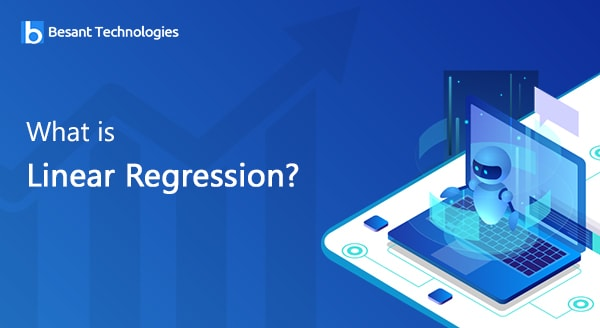# What is Linear Regression?

## What is Linear Regression?

In this topic we actually learn how do we make Machines learn from data and what particular Mathematics is used behind that and how to the particular algorithm is built.

Linear Regression is a part of Supervised Learning Technique which is first most basic part of Machine Learning. So, Linear Regression can be called as first most Machine Learning algorithm.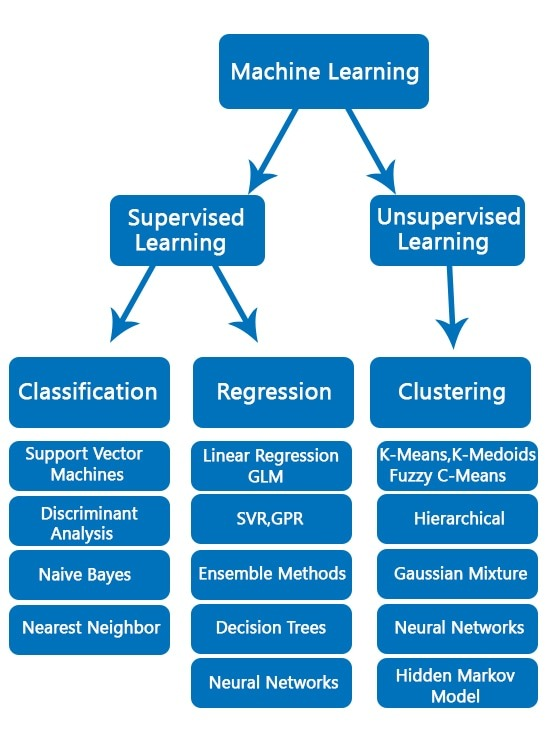### Definition:

Linear Regression is a Supervised Learning Algorithm that learns from a set of training samples. It estimates the relationship between a dependent variable (target/label) and one or more independent variable (predictors).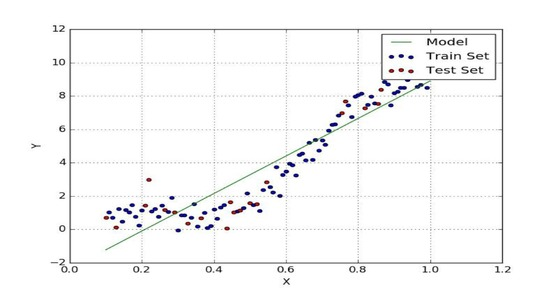Regression Equation:

y = mx + c

Where,

Y is Output variable,

X is Input variable

m and c are the parameters### Types of Linear Regression:

There are three types of Linear Regression. They are:

1. Univariate Linear Regression
2. Multivariate Linear Regression
3. Polynomial Linear Regression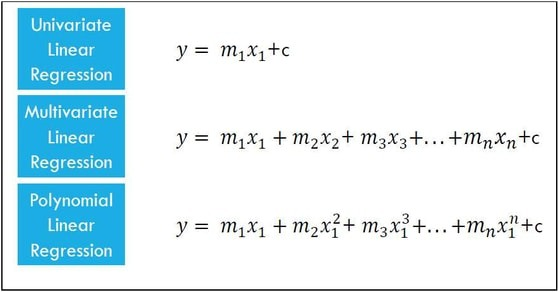So, clearly from the equation we can make out that the Regression equation depends on the values of m and c. Let’s see that in the below examples.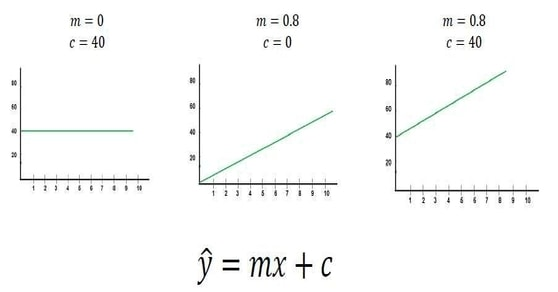is the predicted value

When m=0 and c=40; Line is parallel to the X-axis.

When m=0.8 and c=0; Line passes across center.

When m=0.8 and c>0; Line is shifted above the x-axis for the value equal to c.

#### Error function:

e =  – y

Where is Predicted value and

Y is Actual value.

Error depends on the values of m and c.Our aim here is to build an algorithm which can minimize the error of the problem.#### Cost function:

For that we use the cost function of Linear Regression. Which is,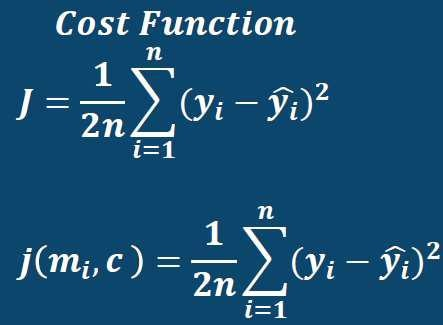Here goal is to minimize the cost function J by changing m and c. This is called optimization and in order to perform this we need particular optimizers. The most important optimizer we use here in Linear Regression among all is Gradient Descent Algorithm.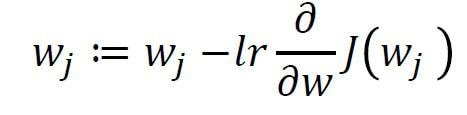Where,

w is the parameter(m or c) and

lr is the Learning rate

### What is Learning Rate?

Learning Rate is the value which the developer (person building the model) gives which decides how fast the algorithm learns from data.

Learning Rate should range between 0 to 1.

Simple Python code for Linear Regression:

```# Importing Libraries
import numpy
import matplotlibas plt
Import pandas
# Importing data set